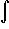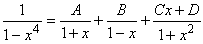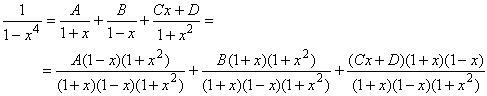#Interactive Real Analysis

Next | Previous | Glossary | Map

## 7.2. Integration Techniques

### Example 7.2.12(b): Integrating Rational Functions

Find the integral(1 - x4)-1 dx
We want to again use partial fraction decomposition, so we need to factor the polynomial p(x) = 1 - x4. Clearly:
1 - x4 = (1 - x2) (1 + x2) = (1 + x) (1 - x) (1 + x2)
By the partial fraction decomposition theorem we therefore know that:We can again combine the right side as follows:so that
1 = A (1-x)(1+x2) + B(1+x)(1+x2) + (Cx+D)(1-x2) =
= A + Ax2 - Ax - Ax3 + B + Bx2 + Bx + Bx3) + Cx - Cx3 + D - Dx2 =
= (A + B + D) + x(-A + B + C) + x2(A + B - D) + x3(-A + B - C)
for all x. This gives four equations in four unknowns:
 A + B + D= 1 (for the constant coefficient) -A + B + C = 0 (for the x coefficient) A + B - D = 0 (for the x2 coefficient) -A + B - C = 0 (for the x3 coefficient)
which - perhaps after reviewing how to efficiently solve systems of linear equations - gives the answer:
A = 1/4, B = 1/4, D = 1/2, C = 0
Therefore we can solve our original integralNext | Previous | Glossary | Map